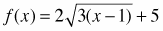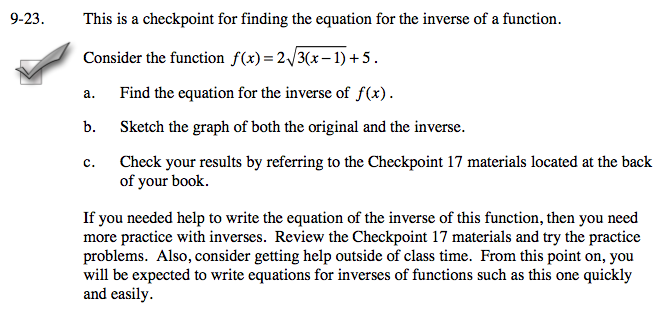### Home > A2C > Chapter 9 > Lesson 9.1.1 > Problem9-23

9-23.
1. This is a checkpoint for finding the equation for the inverse of a function. Homework Help ✎Consider the function.

1. Find the equation for the inverse of f(x).

2. Sketch the graph of both the original and the inverse.

3. Check your results by referring to the Checkpoint 17 materials located at the back of your book.

If you needed help to write the equation of the inverse of this function, then you need more practice with inverses. Review the Checkpoint 17 materials and try the practice problems. Also, consider getting help outside of class time. From this point on, you will be expected to write equations for inverses of functions such as this one quickly and easily.Answers and extra practice are located in the back of your printed textbook or in the Reference Tab of your eBook.
If you have an eBook for A2C , login and then click the following link: Checkpoint 17 Answers and Practice